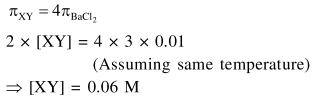# The osmotic pressure of a dilute solution of an ionic compound

Question:

The osmotic pressure of a dilute solution of an ionic compound $\mathrm{XY}$ in water is four times that of a solution of $0.01 \mathrm{M} \mathrm{BaCl}_{2}$ in water. Assuming complete dissociation of the given ionic compounds in water, the concentration of $X Y$ (in $\mathrm{mol} \mathrm{L}^{-1}$ ) in solution is :

1. $6 \times 10^{-2}$

2. $4 \times 10^{-4}$

3. $16 \times 10^{-4}$

4. $4 \times 10^{-2}$

Correct Option: 1

Solution: